# Feature engineering & EDA

Thirumala Reddy

### Last Updated

December 16, 2022

Seclect Blog CategorySTEPS IN DATA PREPROCESSING:-

2. Handle Nulls in the dataset
3. Handling categorical columns
4. Handle Outliers
5. Handle Imbalanced data
6. Feature Selection

• Info, Nulls, Describe
• Check value_counts for some columns you doubt (categorical)
1. Handle Nulls in the dataset
•  Remove Rows having Nulls (losing data)

In most cases removing the null values is not preferred. If we have millions of records & in that only less number of records are missing we can remove the null values.

• Fill Values - mean, median, mode, ffill, bfill

The best way of dealing with null values is by filling in the null values. Depending on the type of data we have we can fill the null values by using mean, median, mode, ffill & bfill.

--> Let's see some of the use cases to fill null values:-

1. When we have an age column with null values fill the null value with the mean or median.
2. If we have categorical data we can fill the null values with mode
3. We have a stock value of a particular product of 1/05/2022,2/05/2022 & 4/05/2022. The stock value of 3/05/2022 is missing in this condition it is always suggestible to fill the stock value of 3/05/2022 by using forward filling(ffill) i.e. in forward filling the stock value of 2/05/2022 is filled for 3/05/2022.
4. Similarly in Backward filling, we fill the stock value of 3/05/2022 is filled with the value of 4/05/2022.
5. The major drawback in the forward filling is if the first row has null values means the null values will be maintained throughout the data & vice-versa with backward filling.

-->    After filling in the null values with any of the methods, plot a graph between the old values i.e. before filling in the null values & the new values i.e. after filling in the null values. This will help us to find the change in the distribution of data before & after filling in the null values.

1. Mean/Median Imputation is easy to implement.
2. Median Imputation is not affected by outliers.

1. Mean/Median Imputation makes changes in the original variance
2. Mean/Median Imputation Impacts the correlation
• Machine learning ways

By using the machine learning models such as KNN & Iterative Imputer methods also we can fill the null value

3. Handling categorical columns

Let's discuss types of Encoding. There are two types of encoding

1) Nominal Encoding

2) Ordinal Encoding

1)Nominal Encoding:-

Nominal encoding is nothing but the features where variables have no order or rank to this variable's feature.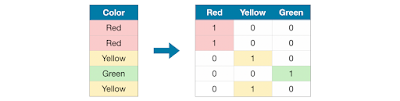-->The different types of Nominal Encoding are

1. One Hot Encoding
2. One Hot Encoding with many categorical
3. Mean Coding

Among these, all Nominal Encoding One Hot Encoding is preferred.

1. One Hot Encoder

If categories > 2 & <7 use One Hot Encoder. By using a Label Encoder for the dataset which more than 2 categories there is a chance of High Bias so by using One hot encoder we can remove the bias of higher numbers.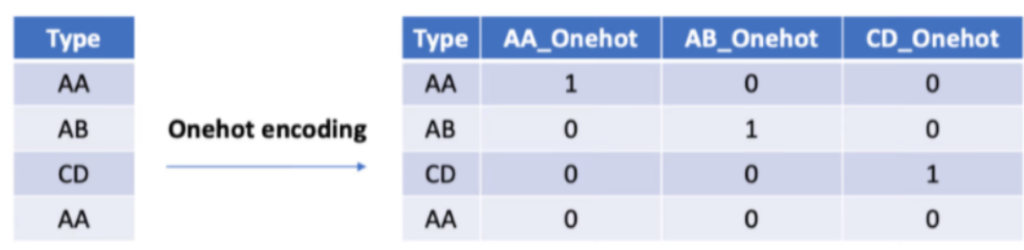2. Ordinal Encoder

Ordinal Encoding is nothing but the feature where variables have some order or rank.

1. Label Encoding
2. Target guided ordinal Encoding.

Label Encoding

Label Encoder assigns a unique number (starting from 0) to each category.

-->If we have nan values present in the data & after doing Label Encoding, the nan value also will be classified into separate categories.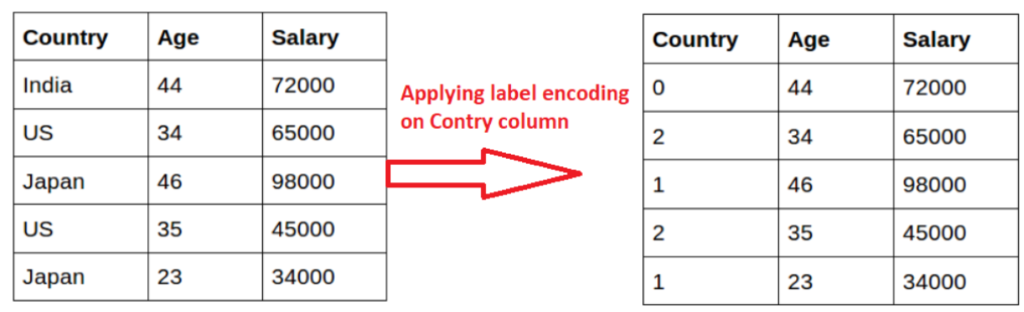1)Straightforward to implement

2)Does not require hours of variable exploration

3)Does not expand massively the feature space(no of columns in the dataset)

1)Does not add any information that may make the variables more predictive

2)Does not keep the information of the ignored labels

Click here to see how to apply one hot encoder & Label Encoder

-->We can handle categorical columns by using a library present in pandas i.e. get_dummies

Click here to know how to apply get_dummies to handle categorical features

4. Handle Outlier

a. Remove outlier (Not recommended)

b. Clip

c. Make outliers as Nulls, and do Fill in Missing

Which Machine Learning Models Are Sensitive To Outliers?

1. Naïve Bayes Classifier---------------------Not Sensitive To Outliers
2. SVM-------------------------------Not Sensitive To Outliers
3. Linear Regression-----------------Sensitive To Outliers
4. Logistic Regression---------------Sensitive To Outliers
5. Decision Tree Regressor or Classifier----Not Sensitive
6. Ensemble(RF,XGboost,GB)-----------Not Sensitive
7. KNN-------------------------------Not Sensitive
8. K Means---------------------------Sensitive
9. Hierarchal------------------------Sensitive
10. PCA------------------------------Sensitive
11. Neural Networks------------------Sensitive

How to find out the outliers:-

1)By Using Box plot:-

We can find the outliers by using a box plot. We can consider the values as outliers if they are less than the minimum value & greater than the maximum value from the Box plot

2)By Using Z-Score: -
The values which are less than the mean- 3*std and greeter than the mean+ 3*std are treated as outliers.
3)By Using IQR: -
If the values are greater than the upper quartile and less than the lower quartile, then those values are treated as outliers.IQR=Inter Quartile Range=Q3 - Q1
Upper Quartile=Q3 + 1.5*IQR
Lower Quartile=Q1 - 1.5*IQR
-->By all the above methods, we can find out outliers.
-->Now we can handle the outliers by removing them, but it results in data loss. So, removing the outliers is not preferred.
-->Now let's take a look at another method to handle outliers i.e. by using KNN IMPUTER.
KNN IMPUTER: -
-->In this method first we make the outliers as nan values & then we fill the nan values by using KNN Imputer.
-->KNN Imputer works on the concept of distance i.e. KNN Imputer fills the nan value with the nearest value present in the dataset. The distance is calculated by using the Euclidian distance or Manhattan distance.

5. Feature Selection

a. Manual Analysis

b. Univariate Selection

c. Feature Importance

d. Correlation Matrix with Heatmap

e. PCA (Principle component analysis)

-->For selecting the features manually we will take the help of domain exports.

Ex: - While solving Banking domain problem statements we will take the help of banking domain people for selecting the features.

Univariate selection:-

In the univariate selection, we use the SelectKBest library present inside learn. SelectKBest internally applies the chi-square test and gives the out chi-square score. Based on this we will select the top features.

Click here to see how to select features by using the univariate selection

Correlation Matrix with Heatmap

In this, we construct the correlation matrix with a heatmap, and from the heat map, we can get what are the features that are more important for predicting the output.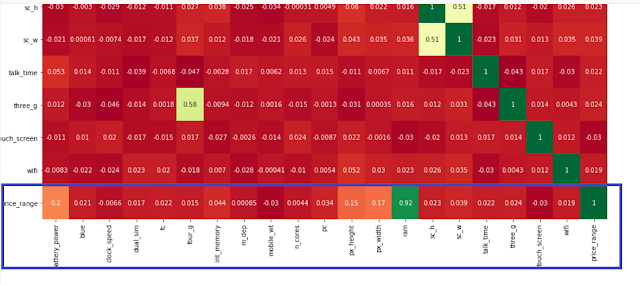-->From the above heatmap, we can observe that the price_range is the output columns & with respect to output columns ram has the highest correlation value of 0.92 next is battery power, etc.

Click here to see how to select features by using a correlation matrix with a heatmap

6. Scale your data (normalize data in a certain range)

MinMax Scaler, Standard Scaler, Robust Scaler

Scaling helps to bring all Columns into a Particular Range

1)MinMax Scaler: -

MinMax scaler converts the data between 0 & 1 by using the min-max formula.

-->Below is the formula of the min-max scaler.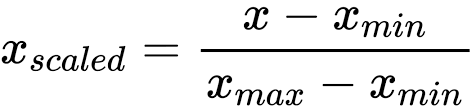2)Standard Scaler:-
The standard scaler converts the data values such that mean=0 & standard deviation=1.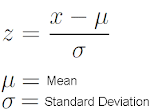3)Robust Scaler: -

Robust Scaler is used to scale the feature to median and quantiles Scaling using median and quantiles consists of subtracting the median from all the observations and then dividing by the interquartile difference.

IQR = 75th quantile - 25th quantile

X_scaled = (X - X.median) / IQR

Some machine learning algorithms like linear and logistic assume that the features are normally distributed

If the data is not normally distributed follow the below steps

- logarithmic transformation

- reciprocal transformation

- square root transformation

- exponential transformation (more general, you can use any exponent)

- box cox transformation

Refer to this for all the above transformation technique implementation

Which  Models require Scaling of the data?

1)Linear Regression-->Require

2)Logistic Regression-->Require

3)Decision Tree-->Not Require

4)Random Forest-->Not Require

5)XG Boost-->Not Require

6)KNN-->Require

7)K-Means-->Require

8)ANN-->Require

i.e. distance-based models & the models which use the concept of Gradient Descent require Scaling.

-->fit_transform is applied only on the training dataset & on the testing dataset only transform is used, this is done to avoid data leakage.

Let's Discuss some of the Automated EDA Library

There are different kinds of Automated EDA Library.

-->Some of them are

1)DTale

2)Pandas Profiling

3)Seeetviz

4)autoviz

5)DataPrep

6)Pandas Visual Analysis